## 7210 Reputation

10 years, 200 days

## questions on mint and differences from m...

Maple 2022

I use the command line mint  since all my code in .mpl files.

First question:

I noticed mint complains that module name is global, for proc inside the module itself, when the name is used as type of a local variable. But maplemint does not complain. Here is an example

```A:=module()
local B:=module()
option object;
export n::integer:=1;
end module;

export foo:=proc()
local a::A:-B;  #mint complains that A is global not declared!
a:=Object(A:-B);
a:-n:=2;
end proc;
end module;```

running mint -i 2 A.mpl gives

These names were used as global names but were not declared:  A

But A is the module name where the whole code is sitting inside it?  To fix this, I have to add

```A:=module()
local B:=module()
option object;
export n::integer:=1;
end module;

export foo:=proc()
local a::A:-B;
a:=Object(A:-B);
a:-n:=2;
end proc;
end module;
```

And now the message/warning is gone. But the above looks really strange.  maplemint does not complain about global A: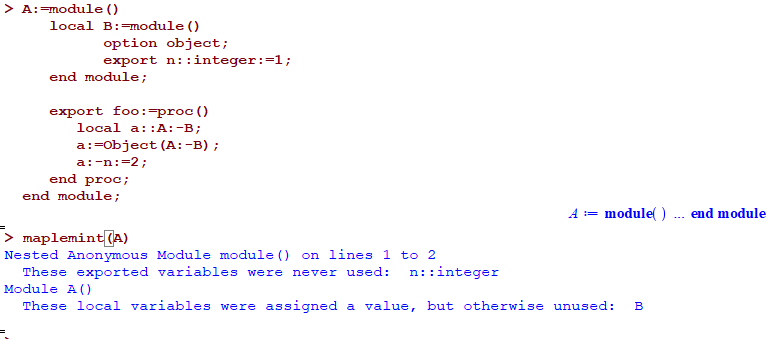But notice that maplemint gives waring that n is never used but mint do not. Another difference!

Which is right about the global name message?

Second question is: I do not understand the message

These local variables were assigned a value, but otherwise unused:  B

which both mint and maplemint give,

What does it mean B is not used?? I used it to make object a inside proc foo().

What would one do to get rid of this message?

Final comment:

I find many message come out that are not real problems at all. For example, if I declare local variable and use it in equation, as symbol, mint complains that the variable was not assigned a value. Here is an example

```foo:=proc()
local x,eq;
eq := x^2;
return eq;
end proc;
```

now maplemint(foo) gives

Procedure foo()
These local variables were used but never assigned a value:  x

I know there are ways to filter out these messages. But I am afraid if I do that, I will filter out a message that indicates a real problem?

According to help   -i 2 shows Display severe and serious errors (default)

if I use -i 1, then these message do not show. What do others use?  Level 1 or 2? If I use level 3, then more strange messages show which I do not understand at all how to remove. Such as

These parameters or local variables are also system defined names:
thismodule
These names are special to Maple:
thismodule

So I stick to level 2 for now. but 90% of the messages I get are not real errors at all.

## different result for loop on expr if it...

Maple 2022

When running this

```expr:=5;
for item in expr do
print(item);
od;```

it prints 5 as expected. When when running

```expr:=I;  (* NOTICE this is complex  I not the number 1 *)
for item in expr do
print(item);
od;
```

it prints the number 1 and not I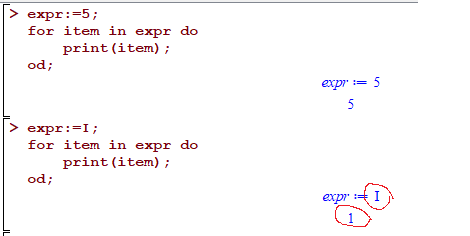I am sure there is a good reason why this happens.

But what should one do to insure they get the complex I in the second example when iterating over a sum of numbers, which in this example happened to be just one number who is complex? I know I can add explicit check to avoid this edge case.

The problem is that I want to iterate of sum of iterms, One or more of them can be complex I. (it is a result of doing series expansion of function at infinity, and I want to iterate over each term in the series one by one).

```expr:=9+I:
for item in expr do
print(item);
od;
```

Gives   9,1   and not 9,I

While

```expr:=a+I:
for item in expr do
print(item);
od;
```

does now give exected output   I,a  and not 1,a

What should one do to insure the for loop always get each term in the sum, even if it is complex?

I can't check the item inside the loop, because by then it is too late as the compelx I is lost already.

Is there a better way to iterate over sum of terms and look at each term as is and not lose the complex I in the way?

Maple 2022.1

Update

For now, I am doing this hack. Since the input is always a sum (with + or - terms), then I convert the input to string, split on delimitors and then parse the entries back to Maple and now it is a list so I can iterate over each term.

```expr:=I+3:
String(expr):
StringTools:-SubstituteAll(%,"+","|+"):
StringTools:-SubstituteAll(%,"-","|-"):
StringTools:-Split(%,"|"):
map(Z->parse(Z),%):
for item in % do
print(item);
od;
```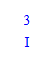And when expr is

```expr:=1+5*I+sin(x)-sqrt(8)*I-a;
```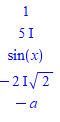It is a hack, but I could not find a relaible way to iterate over each term in the sum of terms without losing the complex I if one term was complex. I need to test this more. It is meant to work only on expression which is sum of terms, which is where I will use it.

Compare the output of the above for expr:=1-I;

The for loop gives 1,-1  but the string hack gives 1,-I which is what I wanted.

Ofcourse the above could fail if there is a "-" or "+" inside the term itself (for example  1+I+sin(1-x) , but this do not happen for the cases I am using this for, which just looking at terms of series expansion around either zero or infinity. These will be just power series terms separated by "+" or possibly "-"

If there is better way do this, that will be great.

## recommended way not make proc return bac...

Maple 2022

Given a module A, it has a proc which is called to set some internal variable to some value. This proc is meant to be used as initialization of the module, and not meant to return anything (i.e. procedure vs. a function in other languages, where a procedure does not return anything, but a function does).

When this proc is called from outside, the result of the last assignment in the proc is returned back to the user since that is the default behavior.

Is there a way to prevent this, other than adding an explicit NULL at the end of this proc?  Adding : at the end the last statement or at the end of the proc did not prevent this.

Here is an example

```restart;
A:=module()
local r::integer;
export set_r:=proc(r::integer)
A:-r:= r:
end proc:
end module:
```

Now when calling  A:-set_r(10), Maple will return back/echo back 10. But this is not something I want.

`A:-set_r(10);`

10

To prevent this, currently I add NULL; at end of the proc, like this

```A:=module()
local r::integer;
export set_r:=proc(r::integer)
A:-r:= r:
NULL;
end proc:
end module:
```

and now

`A:-set_r(10);`

returns nothing. Well, it returns NULL but that is like nothing.

My question is, is there a better way to do this? Can one define a proc in Maple that returns nothing?

Maple 2022.1

## question on using structured type, anyfu...

Maple 2022

I want to replace   expression that contains function whose first argument is always R0 with r0.

I can do it when the function is  f(R0) or f(R0,x) but I do not know how to tell Maple to do the replacement of the first argument, if the function has 0 or more arguments beyond R0.  This is what I tried

 > restart;
 > #this works expr:=A+f(R0); evalindets(expr, 'anyfunc(identical(R0))', Z-> subsop(1 = r0, Z ));> #this also works, when there is one argument after R0 expr:=A+f(R0,x); evalindets(expr, 'anyfunc(identical(R0),anything)', Z-> subsop(1 = r0, Z ));> #but how to do it for any number of arguments after R0? This does not work expr:=A+f(R0,x,y); evalindets(expr, 'anyfunc(identical(R0),anything)', Z-> subsop(1 = r0, Z ));> #I do not want to keep adding anything to match the number of argument, as I do not know how many there could be expr:=A+f(R0,x,y); evalindets(expr, 'anyfunc(identical(R0),anything,anything)', Z-> subsop(1 = r0, Z ));>

I do not want to keep writing anything,anything,anything, for as many times as there could be more arguments.  I wan to say something like

evalindets(expr, 'anyfunc(identical(R0),anything_zero_or_more_arguments)', Z-> subsop(1 = r0, Z ));

Is there a better way to do this whole thing than what I am doing?

Update

Just after I posted this question, I found another structured type that worked

```expr:=A+f(R0,x,y,z,h);
evalindets(expr, 'patfunc(identical(R0),anything)', Z-> subsop(1 = r0, Z ));
```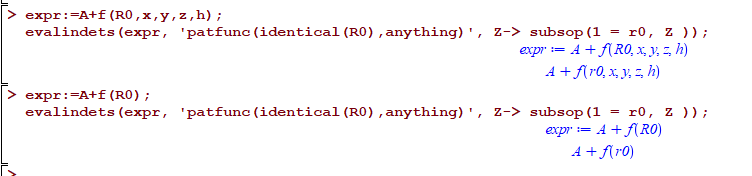So patfunc works for zero or more argument after R0, which is what I wanted.

Given that types are core part of Maple, I find it so amazing that the help page "Definition of a Structured Type in Maple"  has so little examples and description of all these types in it. I can't figure what 80% of the types there do, since there are no examples.

Maplesoft should really do a better job on this page and add much much more examples and description. After all, strong typing is what is supposed to be the strength of Maple. But looking at this help page, it does not give one this impression at all.

## how to obtain this simplification in Map...

Maple

I want to solve for y in   exp(sqrt(y))= tanh(x) and get y=ln( tanh(x)^2).  But unable to figure what options or assumptions Maple needs.

For reference, here is Mathematica solution that I'd like to duplicate in Maple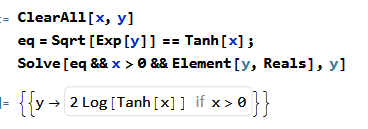Here is my Maple attempts, which uses same assumptions, but Maple solution converts the tanh(x) into exponentials which is much more complicated to look at. I tried to also simplify the answer to tanh(x) but no success so far.

Any ideas what else to try for solve?

I know that PDEtools:-Solve does the job. Which is why I find this strange. I thought that both PDEtools:-Solve and solve end up using same core code at one point. May be I should switch all my code to use PDEtools:-Solve?  Should not solve here have given same answer as PDEtools:-Solve?

Maple 2022.1 on windows 10.

 > eq:=sqrt(exp(y))=tanh(x);> sol:=solve(eq,y) assuming y::real, x>0> simplify(sol)  assuming real, x>0; convert(%,trigh)> sol:=PDEtools:-Solve(eq,y) assuming y::real, x>0>